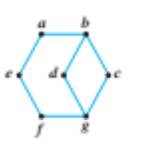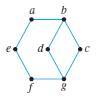Chapter 10.1, Problem 34ES### Discrete Mathematics With Applicat...

5th Edition
EPP + 1 other
ISBN: 9781337694193

#### Solutions

Chapter
Section### Discrete Mathematics With Applicat...

5th Edition
EPP + 1 other
ISBN: 9781337694193
Textbook Problem
1 views

# In 34-37, find Hamiltonian circuits for those graphs that have them. Explain why the other graphs do not.To determine

Find Hamiltonian circuits for those graphs that have them. Explain why the other graphs do not.Explanation

Given information:

Calculation:

Given a graph G, from the subgraph H consisting all vertices of degree 2 and their incident edges.

If G has a Hamiltonian circuit H (which we consider as a subgraph of G ), then it must contain H’ as a subgraph, since a Hamiltonian circuit must include every edge incident on a vertex of degree 2.

If H contains a vertex with degree > 2, then G has no Hamiltonian circuit.

If H has a vertex of degree > 2, then so does H, which is impossible since H is a circuit.

If H contains a circuit, but V(H)V(G), then G has no Hamiltonian circuit

### Still sussing out bartleby?

Check out a sample textbook solution.

See a sample solution

#### The Solution to Your Study Problems

Bartleby provides explanations to thousands of textbook problems written by our experts, many with advanced degrees!

Get Started